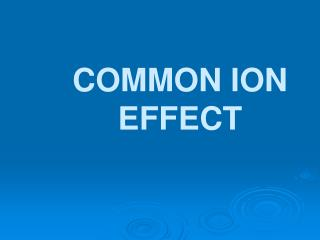DownloadDownload PresentationCOMMON ION EFFECT

# COMMON ION EFFECT

Download Presentation## COMMON ION EFFECT

- - - - - - - - - - - - - - - - - - - - - - - - - - - E N D - - - - - - - - - - - - - - - - - - - - - - - - - - -
##### Presentation Transcript

1. COMMON ION EFFECT

2. COMMON ION an ion common with one in a system at equilibrium which places a stress on the equilibrium Common Ion

3. Uses of Common Ion Effect 1. control pH of a weak acid or base 2. control formation of a precipitate

4. BUFFERExampleNon-example A solution which resists a change in pH when an acid or base is added consists of a weak acid or base and a salt containing a common ion of its conjugate

5. How does LeChatelier’s Principle explain the operation of a buffer?

6. Example of a buffer system CH3COOH + HOH CH3COO-+ H3O+ NaCH3COO(aq)  Na+ + CH3COO-

7. Characteristics of a Good Buffer

8. 1. operates over a narrow pH range (< 1 pH unit) 2. no reactions between buffers in a multiple buffer system 3. range can be extended using more than one buffer

9. Henderson-Hasselbalch Equation

10. 1. What is the pH of a 0.20 M acetic acid solution?

11. Add 10.0 mL of 0.20 M NaOH to 50.0 mL of the preceding solution. What is the pH?

12. Add 5.0g sodium acetate (MM 82.05) to 500. mL of the 0.20 M acetic acid solution. What is the pH?

13. Add 10.0 mL of 0.20 M NaOH to 50.0 mL of the preceding solution. What is the pH?

14. 2. Calculate the mass of ammonium chloride (MM 43.6) needed to buffer 250. mL of 2.0 M ammonia to a pH of 10.

15. TITRATION CURVES

16. Titration Curve A graphical history of a titration typically a plot of the pH (dependent variable) and volume titrant (independent variable)

17. Uses of Titration Curves 1. determine equivalence point 2. determine number of ionization reactions 3. determine optimum buffer region 4. determine possible indicators

18. Shape of Titration Curve Strong acid - strong base Weak acid - strong base

19. Shape of Titration Curve

20. Shape of Titration Curve

21. Equivalence Point 1. Midpoint between points of inflection 2. Plot of the slope of each point of the curve against volume titrant (DpH/DV vs Vavg)

22. Number ofIonization Reactions CH3COOH - NaOH H2C2O4 - NaOH

23. Optimum Buffer Region Area where the concentration of molecules and their conjugate ions are relatively high

24. Indicators Need to choose for each titration system Dependent on pH at equivalence point

25. ACID-BASE INDICATORS

26. Acid-base indicators are weak Bronsted-Lowry compounds that are different colors in acid and base form.

27. Acid-base indicators are all large organic molecules. HIn <===> H+ + In- Color1Color 2

28. Phenolphthalein Colorless acid form, HIn

29. Phenolphthalein Pink base form, In-

30. The color change occurs at a different pH for different indicators. The pH at which the indicator changes color is dependent on the Ka of the indicator as a weak acid.

31. HIn <===> H+ + In-

32. Experiments have shown that the minimum amount of change of HIn <==> In- that can be detected visually is

33. Thus, from the Henderson-Hasselbalch equation, one can select an appropriate indicator for a titration based upon the Ka of the indicator and the pH at the equivalence point.

34. What is the pH at the equivalence point of a titration of 25.0 mL each of 0.10 M HCl and 0.10 M NaOH?

35. What is the pH at the equivalence point of a titration of 25.0 mL each of 0.10 M CH3COOH and 0.10 M NaOH?

36. Phenolphthalein Ka = 1 x 10-9 pH of perceptible color change?

37. SOLUBILITY EQUILIBRIA

38. Saturated Solution Maximum amount of solute dissolved in a specific volume of solvent at a specific temperature

39. Saturated Solution Equilibrium is established between a solid solute and ions from the solute

40. Super-Saturated Solution More than the normal maximum amount of solute is dissolved in a solution.

41. Question at a constant temperature, what is the difference in concentration of a saturated solution: (1 mL vs 1 ML solution) (1 mg vs 1 kg solid)

42. The concentration of a saturated solution remains the same, no matter how much solid is present, as long as the temperature remains constant.

43. The “concentration” of a solid remains the same at a constant temperature.

44. By convention, equations for the formation of saturated solutions are written in the format solid <===> solution AgCl(s) <===> Ag+ + Cl-

45. AgCl(s) <===> Ag+ + Cl-